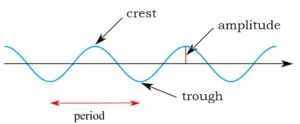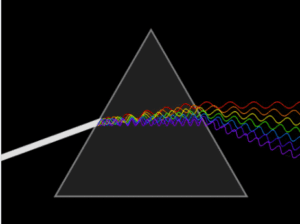Home » frequency and wavelength

# frequency and wavelength

« Back to Glossary Index

Frequency and wavelength are both properties of waves. They are closely related, so this article discusses the two together.

Frequency is the number of repeated motions in a period of time. These motions can be up and down, like a hammer, or back and forth, waving a hand. Or some other repeated motion, maybe the swinging of a pendulum. Repeated motions are called “oscillations” or “cycles.” So, frequency is the number of cycles per unit of time, often measured in seconds.

Cycles can be graphed on paper, as in the accompanying image. In the case of the up and down motion of the hammer, the vertical axis would be elevation and the horizontal axis would be time. When the hammer is highest in elevation, it’s a crest. When it’s lowest in elevation, it’s a trough. Then, over time, the hammer rises again in elevation to a crest, and so on.Key wave properties: period, frequency (number of crests per second), and amplitude. The horizontal axis is time. [Image source: modification of Kraaiennest – CC BY-SA 3.0, https://commons.wikimedia.org/w/index.php?curid=4038226. Retrieved Sept. 21, 2017.]

In this image, frequency is the number of cycles, crest to crest, completed in a second. Or the number of motions, trough to trough—same thing. The more cycles per second, the higher the frequency.

Frequency is symbolized by either the ancient Greek letter v (pronounced nu) or is symbolized simply by f for frequency. The symbol v is generally used for the frequency of electromagnetic waves; whereas, the symbol f is generally used for the frequency of ordinary waves that require a material medium, such as sound and water waves.

The period of the wave is the time lapse between crests (or between troughs). The higher the frequency, the shorter the period. The lower the frequency, the longer the period. Frequency and period are inversely related.

The wavelength is the distance in space from crest to crest. Or from trough to trough—same distance. Wavelength is symbolized by the ancient Greek letter 𝛌, called “lambda.” Like frequency and period, frequency and wavelength are inversely related. The higher the frequency, the shorter the wavelength. The lower the frequency, the longer the wavelength1For electromagnetic waves, which require no material medium, frequency times wavelength equals a constant, the speed of light. This is written mathematically as: c = v𝛌. This means that v .

If you already understand waves but want to know more about frequency and wavelength, you can skip to the section “Wave Motions Occur in Cycles.” If you would like a little more background on waves, read on.

## What is a wave?

A wave is a movement that propagates through a medium. The accompanying animation demonstrates a movement propagating through a crowd in a stadium. The motion ripples along the benches.

The people aren’t moving their bodies down the bench—each sits down again in exactly the same seat. But something is moving around the stadium—a motion—up and then down.

Unlike in physics, the people aren’t physically making each other stand—it’s a mental connection. Each person has decided that when my neighbor stands and then starts to sit, I’ll stand up. So, this is not a wave in the physics definition but is a good metaphor for one.

In a physical wave, a particular motion, an oscillation, is communicated from one bit to the next bit of some medium. It involves a physical push or pull on the neighboring particle. The neighboring particle then pushes or pulls on the next, and so on.

In physics, a wave is a transfer of motion through one end of a medium to the other without matter being transferred. Motion is directed energy, so a wave is a type of energy transfer through a medium.  For more information, see the entry for “wave.”

## Sound Waves—An Example of Frequency and Wavelength

A sound wave is a series of compressions and rarifications (thinning out) of air molecules. These compressions and rarifications push neighboring molecules so that neighbors are physically influenced to repeat the same motion. This creates a propagating wave that travels rhythmically through the air, creating a cycle of low air pressure, and then, high air pressure, and then, low again, as it travels.

The frequency of the sound wave determines its pitch. For example, the frequency of the note that many orchestra instruments tune their instruments to is A above Middle C on the piano. Its frequency is 440 cycles per second. A frequency of 220 cycles per second is the same note, A, but an octave lower.

The accompanying video shows how sound waves work. The section in minutes 1:30 to 4:20 is most relevant, though the entire video is an excellent presentation on sound waves.

## Frequencies of Light Waves—Another Example of Frequency and Wavelength

The frequency of visible light determines its color. Light is made up of electromagnetic waves. As the strengths of the electric and magnetic fields rise and fall, that is, as they oscillate, they create a frequency. The cycle from one crest to the next occurs a certain number of times per second.

The frequency of visible light is measured in “terahertz.” “Tera” is taken from the Greek word for “monster.” And one terahertz is truly monstrous in size: one trillion cycles per second. As shown on the accompanying image, the frequency of visible light ranges from 400 terahertz (red light) to 789 terahertz (violet light).

The frequency of light determines not only to its color, but also, its energy2For waves that require a material medium, for example, sound waves, frequency does not determine energy. For this type of wave, the amplitude (height) of the wave determines its energy. . The higher the frequency, the higher the energy. Violet light, which has about 1.7 times the frequency of red light, also has about 1.6 times the energy. While the relationship between frequency and energy is not one-to-one, it is proportionate. The accompanying table shows the energy levels of each frequency of visible light.

At frequencies lower than red visible light, the nature of electromagnetism changes. These lower frequencies are infrared, microwave, and radio waves. The interactions of visible light with matter causes us to be able to see them. In contrast, the interactions of infrared, microwave, and radio waves with matter causes the matter to heat up.

Above ultraviolet, electromagnetism becomes x-rays, and at even higher frequencies, gamma rays.

X-rays and gamma rays, being higher frequency, also carry higher energies. Their high energies allow them to penetrate matter and also tear apart the atoms and molecules of matter. X-rays and gamma rays can damage our bodies. In fact, gamma rays are among the most damaging forms of radiation emitted by atomic bombs.

The relationship between frequency and energy for electromagnetic waves is expressed mathematically as E = hf, where: E=energy; h = Planck’s constant, an extremely small number3Planck’s constant is .0000000000000000000000000000000006626176. ; and f = frequency. In other words, multiply the frequency of a wave by a tiny number, h, and you will find the wave’s energy.

## Relationship of Frequency, Wavelength, and Speed

Frequency is not the same as the speed of a wave. The speed of a water wave, for example, is the distance the crest of the wave travels in a certain amount of time. Let’s say that we’re watching the crest of the wave coming towards the dock that we’re sitting on. It might travel at 10 miles per hour towards us. But the speed depends on the medium. Substitute molasses for water and the wave would travel a good deal slower. The medium makes all the difference. Frequency and wavelength play no role in determining the speed of water waves and other waves that require a material medium.

The speed of an electromagnetic wave also depends on the medium. But the situation is more complicated than this simple statement. Electromagnetic waves, unlike most waves, do not require a material medium. When an electromagnetic wave travels in a vacuum as opposed to in a material medium, it always travels at 186,282 miles per second. Physicists call all forms of electromagnetism, “light;” 186,282 miles per second is the speed of light.

When an electromagnetic wave travels through a vacuum, frequency and wavelength are related to speed. Specifically, frequency times wavelength equals speed. Wave speed is also sometimes called “velocity.” The relationship between frequency, wavelength, and velocity is expressed mathematically as f ·𝛌 = v. In this equation, f is frequency; 𝛌 is lambda, the Greek letter used to symbolize wavelength; and v is velocity.Prism breaks white light into frequencies. Click on the image for animation. [Image source: By Lucas V. Barbosa – Own work, Public Domain; https://en.wikipedia.org/wiki/Prism; Retrieved May 16, 2018]

Since the speed of light in a vacuum is always the same, how can frequency be related to speed when different electromagnetic waves have different frequencies? Here’s the beauty part—frequency and wavelength compensate for each other because they are exactly inversely related. When multiplied together, they always yield a constant speed, the speed of light.

Electromagnetic waves can also travel through material media, for example, glass. When visible light travels through glass, the waves don’t pass straight through, unaffected. They interact with the glass. Particles of light, photons, are absorbed by the atoms in the glass and are emitted again. Upon being emitted, they resume their path through the glass. Whenever they’re traveling, they’re zipping along at the speed of light. But the stop-start nature of their travel slows them down to an average that is slower than the speed of light.

Let’s say that the light flowing through the glass is white light—a mixture of all the different colors, that is, frequencies. Frequency affects how much the light is slowed down in interactions with the atoms of the glass. So, the different frequencies have different experiences while traveling through glass. If the glass happens to be a prism, it will separate out the frequencies of light according to their speeds. This is why we see white light enter the prism and different colors of light exit the prism.

FOOTNOTES
1For electromagnetic waves, which require no material medium, frequency times wavelength equals a constant, the speed of light. This is written mathematically as: c = v𝛌. This means that v = c/𝛌 and 𝛌 = c/v. Frequency and wavelength are inversely related. For ordinary waves, which require a medium, such as sound or water waves, frequency and wavelength are also inversely related. However, the speed is not a constant and is dependent upon the nature of the medium. If one knows the speed and the frequency, one can find the wavelength with the formula: 𝛌 = s/f, where s = speed and f=frequency. Or, knowing the speed and wavelength, one can find the frequency with the formula: f = s/𝛌.
2For waves that require a material medium, for example, sound waves, frequency does not determine energy. For this type of wave, the amplitude (height) of the wave determines its energy.
3Planck’s constant is .0000000000000000000000000000000006626176.

This site uses Akismet to reduce spam. Learn how your comment data is processed.